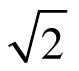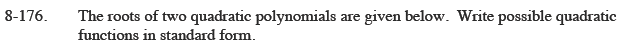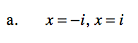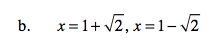Home > CCA2 > Chapter 8 > Lesson 8.3.3 > Problem8-176

8-176.
1. The roots of two quadratic polynomials are given below. Write possible quadratic functions in standard form. Homework Help ✎

1. x = −i, x = i

2. x = 1 +, x = 1 −$y=(x + i)(x - i)$$y=(x - (1 + \sqrt{2}))(x - (1 - \sqrt{2}))$